Skip to content

# MostafaDehghani/bAbI-T2T

The bAbI question-answering dataset ported into T2T.
Latest commit cee1e93 Dec 13, 2018
Type Name Latest commit message Commit time
Failed to load latest commit information.figst2t_usr_dir Nov 1, 2018LICENSE Dec 13, 2018README.md Aug 9, 2018babi_attention.js Jun 25, 2018babi_visualization_UT_act_pondertime.ipynbbabi_visualization_UT_attention.ipynb Jun 25, 2018t2t-babi_decoder Aug 7, 2018

# bAbI-Tensor2Tensor

This repository implements porting the bAbI dataset into Tensor2Tensor framework as well as the Python notebooks for some cool visualizations on how Universal Transformers solve bAbI tasks.

The goal in bAbI tasks is to answer a questions given a number of English sentences that encode potentially multiple supporting facts.

The current existing implementation of bAbI data-generator in Tensor2Tensor is based on the word-level embedding of the input, while the data-generator in this repo supports the fact-level (sentence-level) embedding of the input data as it is used in this paper.

``````@article{dehghani:UT,
author    = {Mostafa Dehghani and Stephan Gouws and
Oriol Vinyals and Jakob Uszkoreit and Łukasz Kaiser},
title     = {Universal Transformers},
year      = {2018},
url       = {https://arxiv.org/abs/1807.03819},
}
``````

Make sure that you have the Tensorflow (preferably V1.9) and Tensor2Tensor installed. Clone the repository in your home directory:

``````cd ~
git clone https://github.com/MostafaDehghani/bAbI-T2T.git
mkdir ~/babi_data
``````

You can download the bAbi data and put it in a temp folder:

``````mkdir ~/babi_data/tmp
cd ~/babi_data/tmp
wget http://www.thespermwhale.com/jaseweston/babi/tasks_1-20_v1-2.tar.gz
``````

Then simply run the following commands to generate the bAbI data for task 1 using the 10k subset:

``````t2t-datagen \
--t2t_usr_dir=~/bAbI-T2T/t2t_usr_dir \
--tmp_dir=~/babi_data/tmp \
--data_dir=~/babi_data/data \
--problem=babi_qa_sentence_task1_10k
``````

You can choose the "task number" from `[0, 20]` and the "subset" from `{1k,10k}` when setting the `--problem`. Using "`0`" as the task number (i.e. `babi_qa_sentence_task0_10k`) gathers test data from all tasks in a single test set in your experiment. Note that this is the join training setup (i.e. over all tasks -> check out the paper for details). For single training, you can use for instance `babi_qa_sentence_single_task1_10k` for task 1, subset 10k.

Since we want to feed the model with sentences, we should take care of this in the beginning of the the `body` functino of the `t2t_model`. So using the existing models in T2T without changing them wouldn't work. Here in this repo, we implemented the modified version of the "Transformer" model and the "Universal Transformer" model. In order to train with one of these models, you can use this command:

``````t2t-trainer \
--t2t_usr_dir=~/bAbI-T2T/t2t_usr_dir \
--tmp_dir=~/babi_data/tmp \
--data_dir=~/babi_data/data \
--output_dir=~/babi_data/output \
--problem=babi_qa_sentence_task1_10k \
--model=babi_transformer \
--hparams_set=transformer_tiny \
--train_steps=100000
``````

Or you can use `--model=babi_transformer` and `--hparams_set=universal_transformer_tiny` to train the fantastic universal transformer on bAbI data. You can change the `--hparams_set` to try different variants of the Universal Transformer, like Adaptive Universal Transoformer.

In order to run the model on test data and decode from the model, you can run the following commands.

``````chmod +x ~/bAbI-T2T/t2t-babi_decoder
~/bAbI-T2T/t2t-babi_decoder \
--t2t_usr_dir=~/bAbI-T2T/t2t_usr_dir \
--tmp_dir=~/babi_data/tmp \
--data_dir=~/babi_data/data \
--output_dir=~/babi_data/output_ut \
--problem=babi_qa_sentence_task1_10k \
--model=babi_universal_transformer \
--hparams_set=universal_transformer_tiny \
--decode_to_file=decoded_ut.txt
``````

This generates a text file for you, containing the records from test dataset with story, question, gold target, and the prediction by the model.

### Universal Transformer on bAbI tasks

Universal Transformer achieves a new state of the art on the bAbI linguistic reasoning task (for detailed results, check the experiments in the paper).

Here, I bring some analysis on this task. As it's explained in our paper to encode the input, we first encode each fact in the story by applying a learned multiplicative positional mask to each word’s embedding, and summing up all embeddings. We embed the question in the same way, and then feed the (Universal) Transformer with these embeddings of the facts and questions. Both the Adaptive and non-adaptive Universal Transformer achieve state-of-the-art results on all tasks in terms of average error and number of failed tasks, in both the 10K and 1K training regime.

To understand the working of the model better, we analyzed both the attention distributions and the average ACT ponder times for this task.

• First, we observe that the attention distributions start out very uniform, but get progressively sharper in later steps around the correct supporting facts that are required to answer each question, which is indeed very similar to how humans would solve the task.

An example from tasks 2: (requiring two supportive facts to solve)

Story:

``````John went to the hallway.
John went back to the bathroom.
John grabbed the milk there.
Sandra went back to the office.
Sandra journeyed to the kitchen.
Sandra got the apple there.
Sandra dropped the apple there.
John dropped the milk.
``````

Question:

``````Where is the milk?
``````

Model's Output:

``````bathroom
``````

Visualization of the attention distributions, when encoding the question: “Where is the milk?”.

Step#1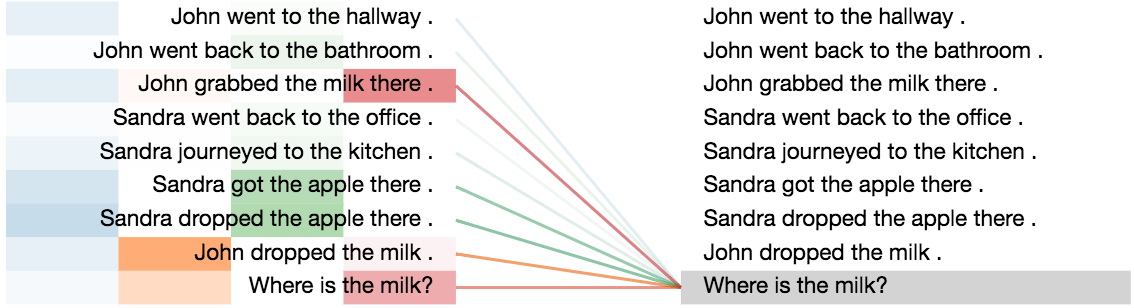Step#2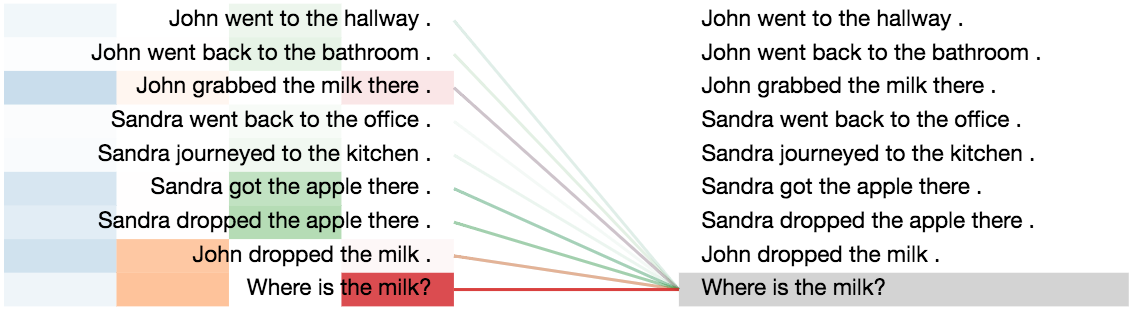Step#3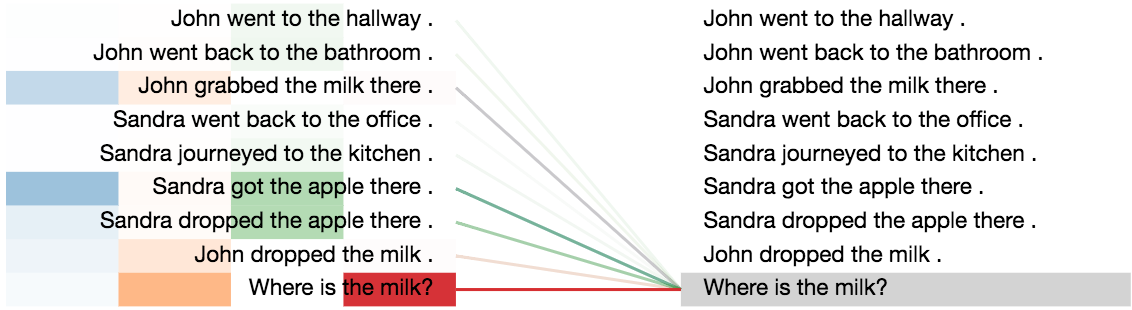Step#4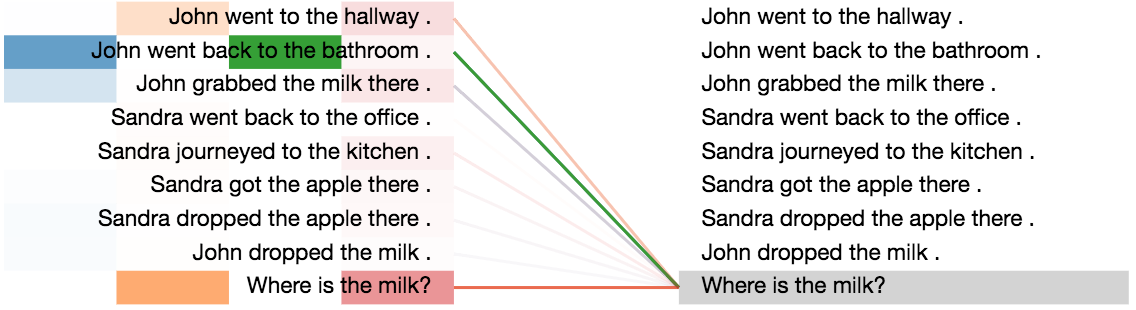• Second, with ACT we observe that the average ponder time (i.e. depth of the per-symbol recurrent processing chain) for tasks requiring three supporting facts is higher than for tasks requiring only two, which is in turn higher than for tasks requiring only one supporting fact.

• Finally, we observe that the histogram of ponder times at different positions is more uniform in tasks requiring only one supporting fact compared to two and three, and likewise for tasks requiring two compared to three. Especially for tasks requiring three supporting facts, many positions halt at step 1 or 2 already and only a few get transformed for more steps. This is particularly interesting as the length of stories is indeed much higher in this setting, with more irrelevant facts which the model seems to successfully learn to ignore in this way.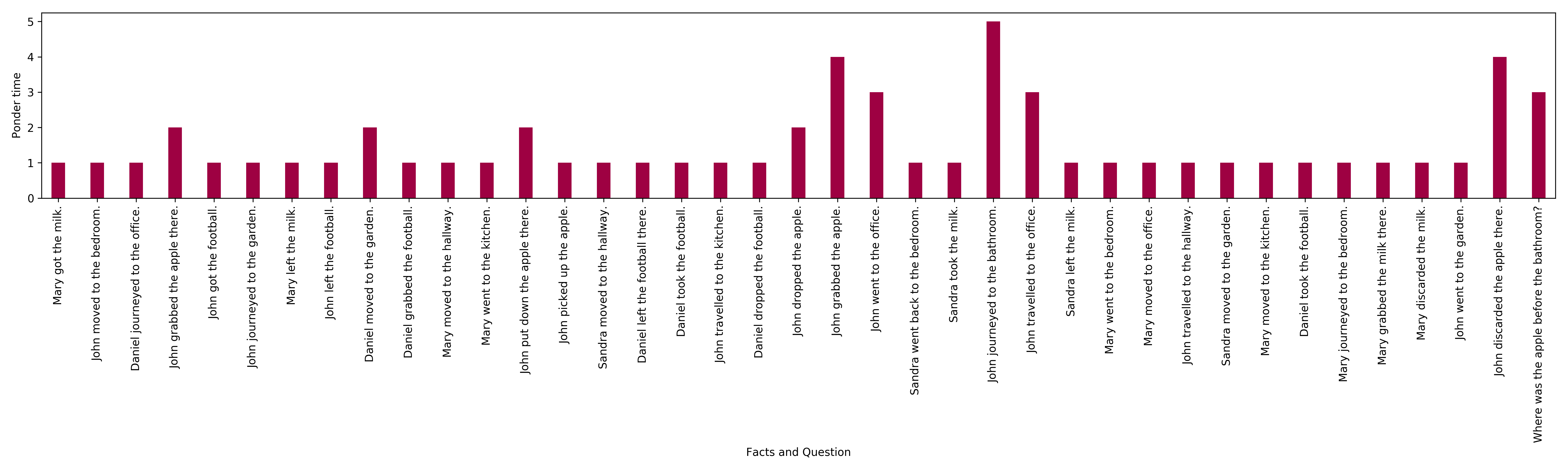The above figure illustrates the ponder time of Adaptive Universal Transformer for encoding facts in a story and question in a bAbI task requiring three supporting facts.

It's worth mentioning that the success of the Universal Transformer (compared to the Transformer model) on bAbI tasks is mainly due to the fact that the Universal Transformer is super data efficient model and is able to support very small datasets.

## TODO:

• Fix and clean the notebook for act pondertime visualization (change based on the new namings, etc.)
You can’t perform that action at this time.